## Quanser QUBE-Servo 2 Pendulum Control Reinforcement Learning

version 1.0.3 (1.63 MB) by
Shows how to design a reinforcement learning agent to balance the Quanser QUBE-Servo 2 Inverted Pendulum.

Updated 19 May 2022

Quanser QUBE-Servo 2 Inverted Pendulum Balance Control using Reinforcement Learning
This example shows how to design a reinforcement learning agent using the MathWorks Reinforcement Learning Toolbox to balance the Quanser QUBE-Servo 2 Inverted Pendulum system, shown below.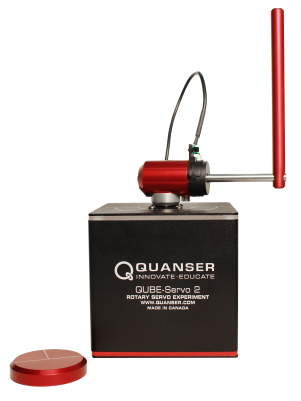This system has two encoders to measure the position of the rotary arm (i.e., the DC motor angle) and the pendulum link and a DC motor at the base of the rotary arm.
How Does it Work?
The Reinforcement Learning (RL) design is discussed in this Quanser blog and includes videos showing the system in action. We also have an eBook version that contains more detail. The agent is trained using the following Simulink model.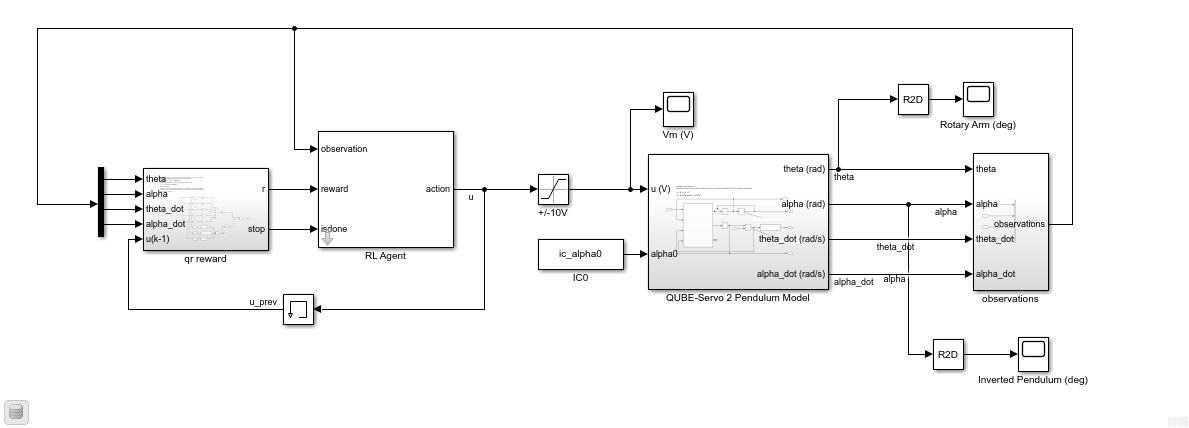The Simulink model uses a nonlinear model of the QUBE-Servo 2 as the RL environment and the RL Agent block to implement the agent. The agent uses the observation and reward signals and outputs an action, i.e., motor voltage, to balance the pendulum.
Before You Run this Example
The pendulum RL balance control can be run using the virtual twin of the QUBE-Servo 2, or the actual hardware. Here are the requirements:
1. MathWorks Reinforcement Learning Toolbox. The RL Agent block is used to train a new agent or load an existing one.
2. Quanser QLabs Virtual QUBE-Servo 2 software. Only needed to run the agent using the QUBE-Servo 2 Virtual Twin. If you don't have QLabs, you can sign up for a free trial here.
3. Quanser QUARC Real-Time Control Software and the MathWorks Deep Learning Toolbox are required to run the agent using the QUBE-Servo 2 hardware. If you don’t have the QUARC software, you can sign up for free trial.
How to Run the Example
1. Select whether you want to load a previously trained agent or train a new one from the drop-down box below. It is recommended that you start by testing the existing agent first.
2. Run each section of the script in sequence up until the Simulate the QUBE-Servo 2 IP RL section.
3. Simulate the RL balance control on the Simulink model shown above as described in that section (e.g., change the initial vertical angle).
4. Go to the Running RL on the Virtual QUBE-Servo 2 IP Experiment section if you want to run the RL balance control agent on the QUBE-Servo 2 virtual twin.
5. Go to the Running RL on the QUBE-Servo 2 IP Hardware section if you want to run RL balance control on the QUBE-Servo 2 hardware.
% Load pre-defined agent (false) or train new agent (true)
doTraining = false;
Simulate RL using the QUBE-Servo 2 Inverted Pendulum Model
This Simulink model that was used in the training can be used to simulate the pendulum balance RL control using the nonlinear rotary pendulum model.
1. Open the s_qube2_bal_rl Simulink model.
3. Try changing in the initial angle of the inverted pendulum somewhere between +/- 10 deg. For example, set the IC0 block to 0.96*ic_alpha0 to have the initial angle start at 172.8 deg, which is 7.2 deg away from the inverted position.
Note: The ic_alpha0 variable is initially set to rad or 180 deg, i.e., the angle of the inverted pendulum when balanced and perfectly upright.
% open Simulink model to simulate QUBE-Servo 2 RL balance control
open("s_qube2_bal_rl.slx");
The responses of the rotary arm and pendulum when the pendulum starts at approximately -7.5 deg from the upright balance position are shown in the Rotary Arm (deg) and Inverted Pendulum (deg) scopes below. The voltage applied to the motor is shown in the Vm (V) scope.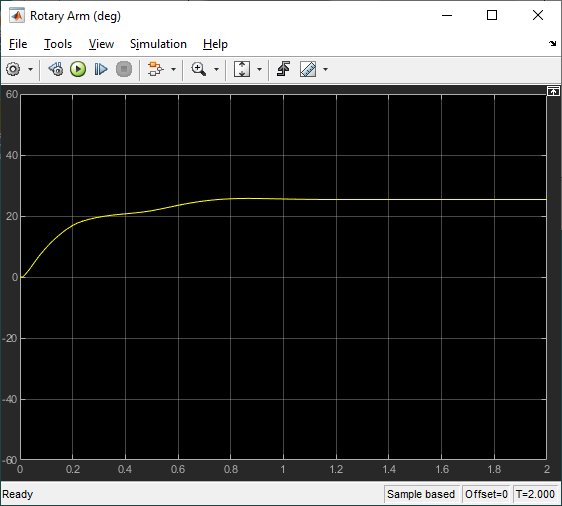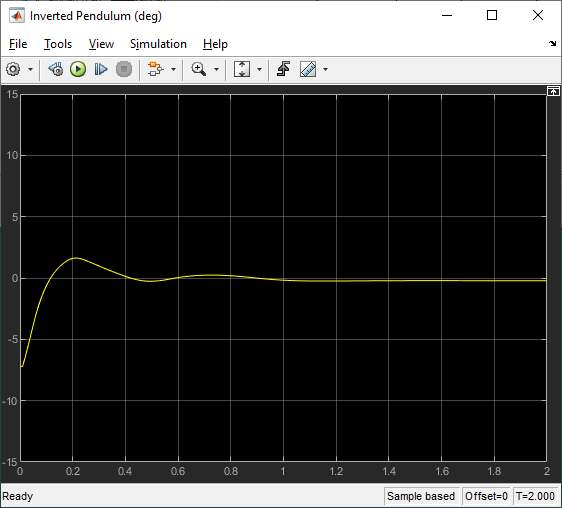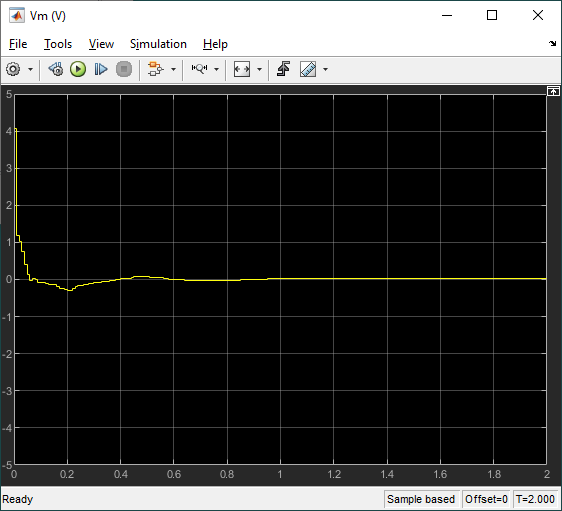Running RL using the Virtual QUBE-Servo 2 IP Experiment
Run the reinforcement learning agent using the QLabs Virtual QUBE-Servo 2 Experiment through Simulink. If you don't have QLabs and want to try it out, you can request for a free trial here.
1. Open the Quanser Interactive Labs (QLabs) software and make sure the Pendulum Workspace in the QUBE 2 - Pendulum menu is loaded as shown above. For more information about running the software, please go to the QLabs support page.
2. Open the Simulink model that interacts with the Virtual QUBE-Servo 2 Pendulum, as shown below.
4. Click on the “Lift pendulum” button in the top-right corner to bring the pendulum up to the inverted position. The RL balance control will engage once the pendulum reaches +/- 10 deg of the vertical.
% open Simulink model to run RL balance control on the Virtual QUBE-Servo 2 pendulum
open("qlabs_qube2_bal_rl.slx");
The scopes below show the response when the system is in steady-state balance mode in the virtual QUBE-Servo 2.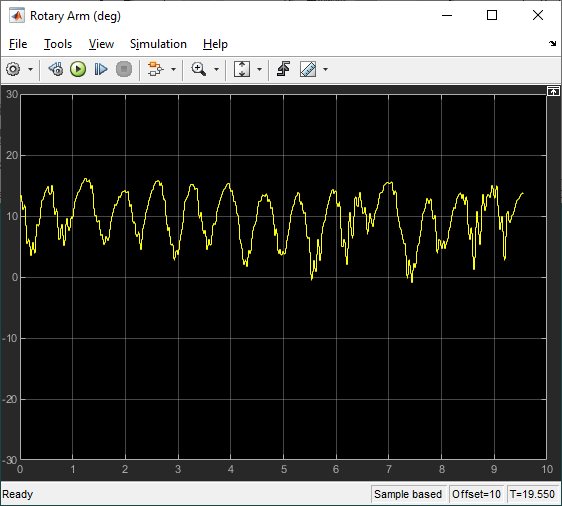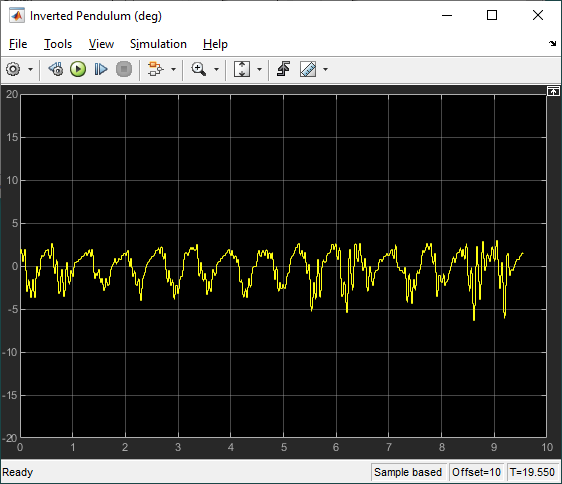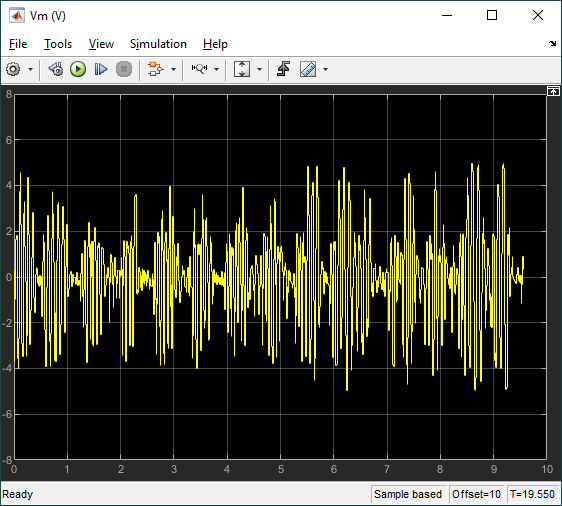Running RL using the QUBE-Servo 2 IP Hardware
The Quanser QUARC Real-Time Control Software is needed to interface to the QUBE-Servo 2 hardware through Simulink. QUARC creates an executable for 64-bit Windows using code generation from Simulink Coder and MATLAB Coder. The QUARC executable is then run through the Simulink interface in full External mode. Currently in R2021a, the RL Agentblock does not support code generation. Instead, the trained agent is deployed on the 64-bit Windows target with QUARC using the Predict block from the Deep Learning Toolbox, as shown below.

Instructions on how to use Simulink and the QUARC Real-Time Control Software to run the RL balance control using the QUBE-Servo 2.
1. Connect the QUBE-Servo 2 to the PC/laptop USB port.
2. Make sure the QUBE-Servo 2 is powered and Power LED is lit.
3. Open the Simulink model that interacts with the QUBE-Servo 2 given below.
4. Click on the "Monitor and Tune" button in the Simulink toolbar to generate and run the QUARC controller.
5. Once the LED is green, manually bring up the pendulum to the vertical position. Immediately release the pendulum once the controller engages (i.e., when it's within +/- 10 deg of vertical).
6. Click on the Stop button to stop running the QUARC controller.
% load the Simulink model that uses QUARC to run the RL balance control on the QUBE-Servo 2
open("q_qube2_bal_rl_hw.slx");
The sample response when the RL policy is deployed and run by QUARC are shown in the scopes below. Here we see an improvement over the Virtual Twin responses. The rotary arm oscillations are smaller, and the pendulum moves less, as it balances about the vertical position. The motor command signal is also smaller, but still has some high-frequency components.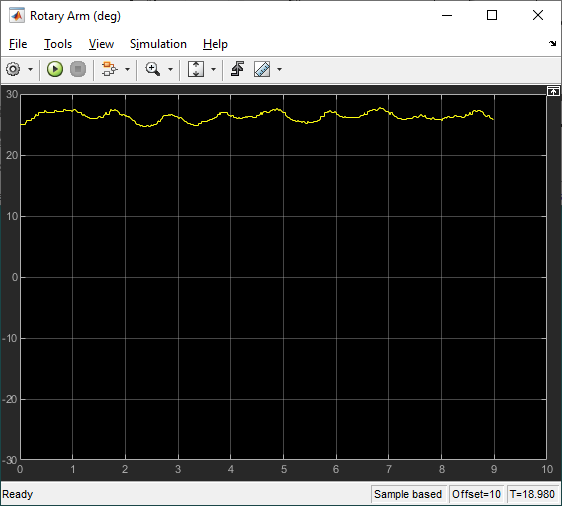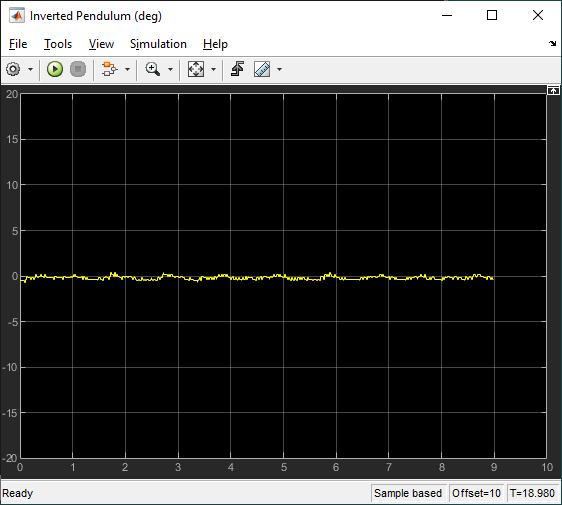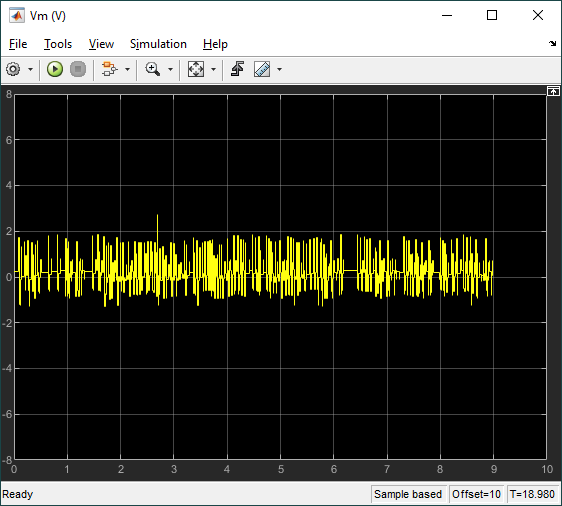Resources

### Cite As

Quanser (2022). Quanser QUBE-Servo 2 Pendulum Control Reinforcement Learning (https://www.mathworks.com/matlabcentral/fileexchange/106935-quanser-qube-servo-2-pendulum-control-reinforcement-learning), MATLAB Central File Exchange. Retrieved .

##### MATLAB Release Compatibility
Created with R2021a
Compatible with R2021a and later releases
##### Platform Compatibility
Windows macOS Linux